# Calculating Dependency

### From basic dependency to inheritance, it's important to know how tightly coupled your code is. Let's trying to bring math into the equation to measure your relationships.

· Java Zone · Tutorial
Save
13.49K Views

what does the concept of dependency mean in programming? is it important in modern development process? does the concept of dependency have a different meaning when we speak about procedural programming, object-oriented programming, or functional programming? in this post, i will try to sum up all the knowledge i gained during my life as a software developer.

## the very beginning

first of all, we need to have, clearly in mind, the concept of dependency in everyday language. merriam-webster gives the following definition of dependency:

the quality or state of being influenced or determined by or subject to another.

clarifying. sometimes we simply need to return to our roots for a deep understanding of a concept. so, a component that is dependent on another is influenced by it. what does that mean? if a component changes internally, its implementation, or externally, its interface, it is probable that all dependent components should change accordingly.

we have just derived that dependency between two components is a measure of the probability that changes to one component could affect also the other . the stronger the dependency between the components, the higher the above probability.

### coupling

coupling between components measures their degree of dependency. tightly coupled components have a high probability of changing together; loosely coupled components have a lower probability.

in software engineering, we have a mantra that was taught to us from the very beginning:

dependency between components must be minimized, making components loose coupled.

why is this principle so important? the main concept behind it is that we should be free to change a component to resolve a bug, or to add a new feature, or to do whatever we want, without having to worry about changes to other components of the architecture.

why are changes considered so dangerous in software development? every time we change a component, there is the risk that we are introducing a bug. to avoid regressions, we have to re-execute all tests associated with the changed components (what if we have not automated testing? ask robert c. martin …)

moreover, we might not directly own the dependent components. in such cases, changes must be discussed with external developers, properly scheduled, and so on. this is one of the main reasons that stops the evolution of an architecture.

finally, this principle is so important because of the dynamism of software architectures. there is no software product that does not change over its lifetime. changes happen, changes are part of the software world.

## object-oriented programming and dependency

in object-oriented programming, the above concept of the component is usually identified by a type . basically, in object-oriented programming, we are interested in dependencies among types — concrete types (classes) or abstract types (abstract classes and interfaces).

the different kinds of dependency between types are well-summarized in the following figure.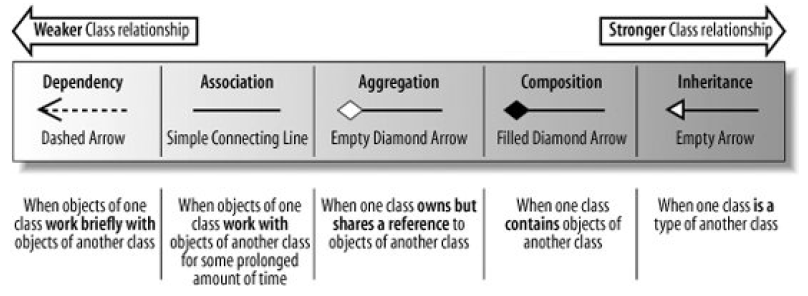the figure maps all possible types dependencies into four equivalence sets. the notation used is uml. let’s have a brief look at every one.

### dependency

on the left, we find the weakest form of dependency. with a dashed arrow between two types in uml, we model a dependency that:

declares that a class needs to know about another class to use objects of that class.

the following code fits exactly the above definition.

``````class a {
def methodofa = ...
}
class b {
def methodwithaparameter(a: a) = ...
def methodwithareturn: a = ...
}``````

as you can see, the only part of ``` a ``` that ``` b ``` needs to know is the interface inferred by ``` a ``` methods. so, if ``` a ``` changes its interface, i.e. the signature of method ``` methoda ``` , a change in ``` b ``` might be required.

### association

increasing the level of dependency, we find association .

association means that a class will actually contain a reference to an object, or objects, of the other class in the form of an attribute.

translating into code, we have something similar to the following.

``````class a {
// ...
}
class b(val a: a) {
// ...
}``````

different from the previous category, an association means that a class is made of references to other classes. their behavior starts to be more coupled because the relationship between the types is not temporary anymore, but it starts to be something permanent (i.e. throughout the lifetime of an object).

in our example, references to ``` a ``` inside ``` b ``` are allowed in every method of the latter, widening the scope of possible dependencies from ``` a ``` .

### aggregation and composition

aggregation and composition are stronger versions of the association relationship: they add additional properties to the latter. essentially, an aggregation states that one type owns the other, which means that it is responsible for its creation and deletion .

the composition adds the feature where if ``` b ``` is a composition of ``` a ``` , then there can be only an instance of the first that owns an instance of the second. instances of ``` a ``` are not shared among different instances of ``` b ``` .

an example of composition is represented by the following code.

``````class b() {
val a: a = new a()
// ...
class a {
// ...
}
}``````

these relationships generate a strong dependence between types because one type has to know how to build an instance of the other.

### inheritance

inheritance represents the strongest type of dependency relationship that can be defined between two different types.

if you find yourself saying that the class is a type of another class, then you might want to consider using inheritance (or generalization).

``````class a(i: int) {
def methodofa = ...
}
class b(i: int) extends a(i) {
def methodofb1 = ...
def methodofb2 = ...
}``````

``` a ``` is known as a parent class , whereas ``` b ``` is known as child class .

a child class inherits and reuses all of the attributes and methods that the parent contains and that have public, protected, or default visibility.

inheritance is also known as implementation reuse . the quantity of code that is shared among the two types is huge. it is possible that every change to ``` a ``` can result in a change to ``` b ``` . this is the real tight coupling ! and did you notice that, using inheritance, the information hiding principle is not respected anymore?

however, not all the types of inheritance are bad guys. inheritance from abstract types mitigates the drawbacks of this kind of relationship. why? the lower the shared code, the lower the degree of dependence. type inheritance , which means that a class implements an interface, it is not harmful: only interface methods’ signatures are shared.

## calculating the degree of dependency

you should have noticed a pattern from the above descriptions. the more the quantity of shared code between two types, the stronger the dependency. it is also true that the wider the scope of this dependency in terms of time, the stronger the dependency.

it would be nice if there was a mathematical formula to calculate the degree of coupling between two classes. with the information that we gave, we can try to formalize it.

given two classes. ``` a ``` and ``` b ``` , the degree of dependency between them can be derived using the following formula.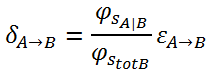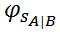is the quantity of code (i.e., sloc) that is shared between types ``` a ``` and ``` b ``` .

is the total amount of code (i.e.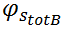sloc) of the ``` b ``` class.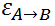is a factor between 0 and 1. the wider the scope between ``` a ``` and ``` b ``` , the greater the factor.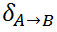here's the result. the values range between 0 and 1: a value of 0 corresponds to no dependency, and a value of 1 corresponds to the maximum degree of dependency.

when ``` a ``` and ``` b ``` hold the weakest type of dependency we saw so far,is near to 0, whereas it is near to 1 when inheritance holds.

for example, if ``` b ``` inherits from ``` a ``` , then, the shared code is represented by all the code of ``` a ``` that does not have a ``` private ``` scope. whereas, if a method of ``` b ``` simply refers to an instance of ``` a ``` among its parameters, the shared code will be represented only by the signatures of ``` public ``` methods of ``` a ``` .

it is important to note that the degree of dependency between ``` a ``` and ``` b ``` it is directly proportional to the probability that if ``` b ``` is changed, ``` a ``` should be changed accordingly.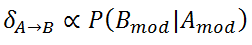finally, the total degree of dependency of type ``` a ``` can be calculated as the mean of the dependency degrees with respect to every other single type in the architecture.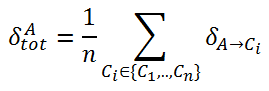## conclusions

summing up, we gave a definition of dependency in software engineering. we tried to understand why dependency among components should be minimized. we revised how uml helps us to visually manage the dependencies, in the object-oriented world. with this information, we tried to formalize a formula to calculate the degree of dependency between two classes.

Topics:
dependency analysis, object oriented programming, java, inheritance, tutorial

Published at DZone with permission of Riccardo Cardin, DZone MVB.

Opinions expressed by DZone contributors are their own.